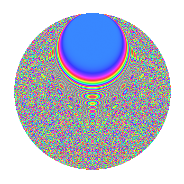# Properties

 Label 3150.2.emLevel 3150 Weight 2 Character orbit em Rep. character $$\chi_{3150}(73,\cdot)$$ Character field $$\Q(\zeta_{60})$$ Dimension 1600 Sturm bound 1440

# Related objects

## Defining parameters

 Level: $$N$$ = $$3150 = 2 \cdot 3^{2} \cdot 5^{2} \cdot 7$$ Weight: $$k$$ = $$2$$ Character orbit: $$[\chi]$$ = 3150.em (of order $$60$$ and degree $$16$$) Character conductor: $$\operatorname{cond}(\chi)$$ = $$175$$ Character field: $$\Q(\zeta_{60})$$ Sturm bound: $$1440$$

## Dimensions

The following table gives the dimensions of various subspaces of $$M_{2}(3150, [\chi])$$.

Total New Old
Modular forms 11776 1600 10176
Cusp forms 11264 1600 9664
Eisenstein series 512 0 512

## Trace form

 $$1600q + 12q^{5} + O(q^{10})$$ $$1600q + 12q^{5} - 12q^{10} - 200q^{16} - 36q^{17} + 72q^{22} + 28q^{23} + 4q^{25} + 8q^{28} - 80q^{29} - 52q^{35} - 20q^{37} - 24q^{38} + 56q^{43} - 12q^{47} - 32q^{50} - 92q^{53} + 24q^{58} - 120q^{59} + 24q^{65} + 144q^{68} - 44q^{70} + 60q^{73} - 144q^{77} + 12q^{80} + 192q^{82} + 104q^{85} - 4q^{88} + 300q^{89} + 24q^{92} + 84q^{95} + 56q^{98} + O(q^{100})$$

## Decomposition of $$S_{2}^{\mathrm{new}}(3150, [\chi])$$ into newform subspaces

The newforms in this space have not yet been added to the LMFDB.

## Decomposition of $$S_{2}^{\mathrm{old}}(3150, [\chi])$$ into lower level spaces

$$S_{2}^{\mathrm{old}}(3150, [\chi]) \cong$$ $$S_{2}^{\mathrm{new}}(175, [\chi])$$$$^{\oplus 6}$$$$\oplus$$$$S_{2}^{\mathrm{new}}(350, [\chi])$$$$^{\oplus 3}$$$$\oplus$$$$S_{2}^{\mathrm{new}}(525, [\chi])$$$$^{\oplus 4}$$$$\oplus$$$$S_{2}^{\mathrm{new}}(1050, [\chi])$$$$^{\oplus 2}$$$$\oplus$$$$S_{2}^{\mathrm{new}}(1575, [\chi])$$$$^{\oplus 2}$$

## Hecke Characteristic Polynomials

There are no characteristic polynomials of Hecke operators in the database